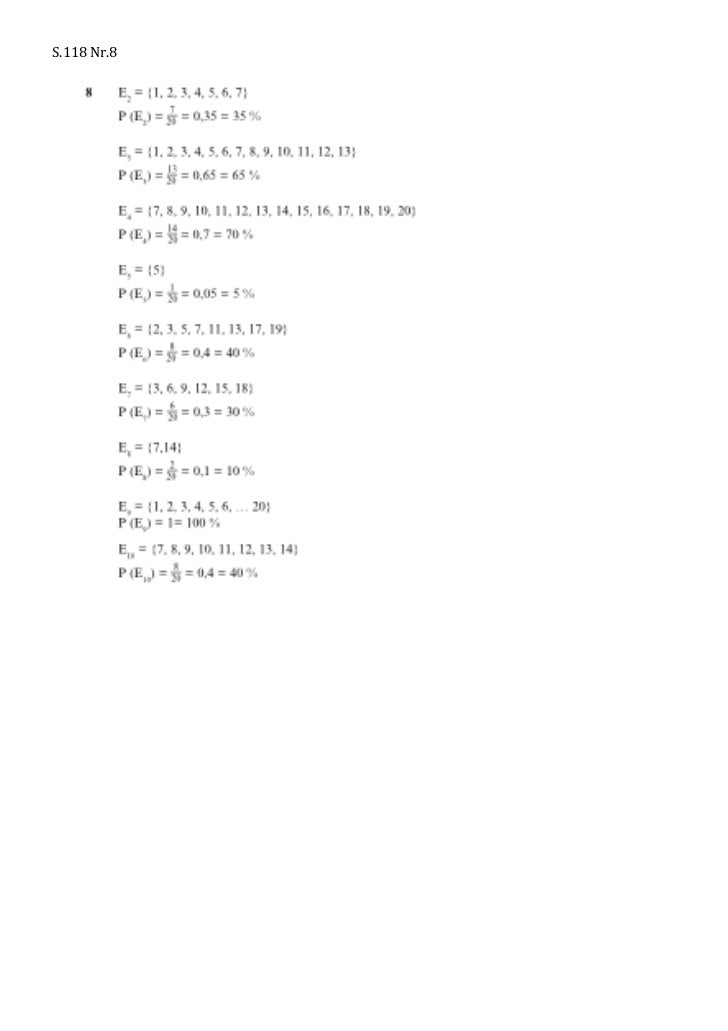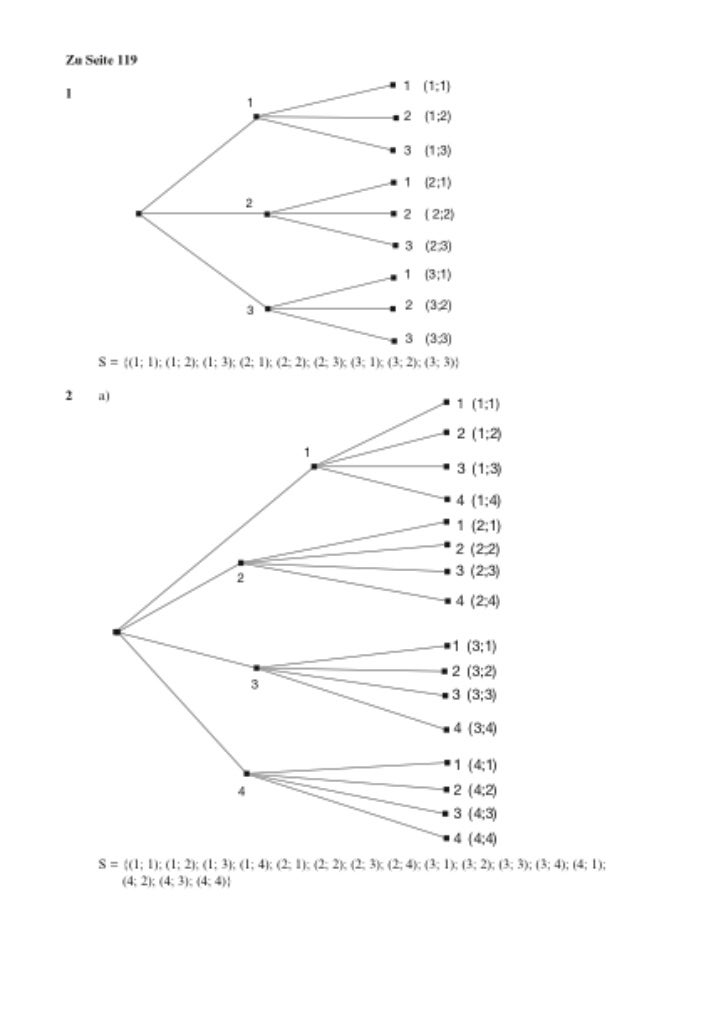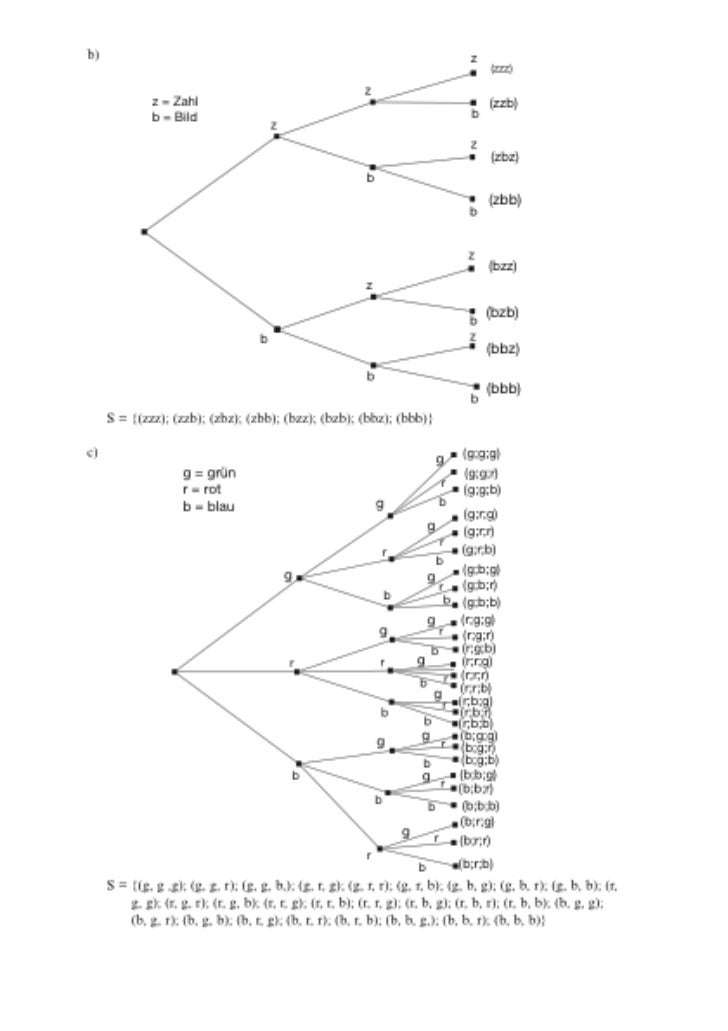Successfully reported this slideshow.Upcoming SlideShare
×

of0

Share

# Lösungen handout

Klasse 8
G S.118 Nr.8
G S.119 Nr. 1+2
G S.127 Nr.1
E S.127 Nr.2
E S. 128 Nr.1+3
G S.120 Nr.1
E S.120 Nr.3
G S.121 Nr.1
E. S.121 Nr.2
Übungsaufgaben S.124 und 125

See all

### Related Audiobooks

#### Free with a 30 day trial from Scribd

See all
• Be the first to like this

### Lösungen handout

1. 1. E3 = {30 €}; P (E3) = 162 = 27 13 P (E4) = P (E2) = 27 102 17S.118  Nr.8   E5 = {15 €, 20 €, 40 €}; P (E5) = 162 = 27   8 E2 = {1, 2, 3, 4, 5, 6, 7} 7 P (E2) = 20 = 0,35 = 35 % E3 = {1, 2, 3, 4, 5, 6, 7, 8, 9, 10, 11, 12, 13} 13 P (E3) = 20 = 0,65 = 65 % E4 = {7, 8, 9, 10, 11, 12, 13, 14, 15, 16, 17, 18, 19, 20} 14 P (E4) = 20 = 0,7 = 70 % E5 = {5} 1 P (E5) = 20 = 0,05 = 5 % E6 = {2, 3, 5, 7, 11, 13, 17, 19} 8 P (E6) = 20 = 0,4 = 40 % E7 = {3, 6, 9, 12, 15, 18} 6 P (E7) = 20 = 0,3 = 30 % E8 = {7,14} 2 P (E8) = 20 = 0,1 = 10 % E9 = {1, 2, 3, 4, 5, 6, … 20} P (E9) = 1= 100 % E10 = {7, 8, 9, 10, 11, 12, 13, 14} 8 P (E10) = 20 = 0,4 = 40 %     93
2. 2. 6 Mit dem Zufall rechnenZu Seite 119 1 (1;1)1 1 2 (1;2) 3 (1;3) 1 (2;1) 2 2 ( 2;2) 3 (2;3) 1 (3;1) 3 2 (3;2) 3 (3;3) S = {(1; 1); (1; 2); (1; 3); (2; 1); (2; 2); (2; 3); (3; 1); (3; 2); (3; 3)}2 a) 1 (1;1) 2 (1;2) 1 3 (1;3) 4 (1;4) 1 (2;1) 2 (2;2) 2 3 (2;3) 4 (2;4) 1 (3;1) 2 (3;2) 3 3 (3;3) 4 (3;4) 1 (4;1) 4 2 (4;2) 3 (4;3) 4 (4;4) S = {(1; 1); (1; 2); (1; 3); (1; 4); (2; 1); (2; 2); (2; 3); (2; 4); (3; 1); (3; 2); (3; 3); (3; 4); (4; 1); (4; 2); (4; 3); (4; 4)}  94
3. 3. 6 Mit dem Zufall rechnen b) z (zzz) z z = Zahl (zzb) b = Bild b z z (zbz) b (zbb) b z (bzz) z (bzb) b b z (bbz) b (bbb) b S = {(zzz); (zzb); (zbz); (zbb); (bzz); (bzb); (bbz); (bbb)} c) g (g;g;g) g = grün (g;g;r) r r = rot (g;g;b) b = blau g b (g;r;g) g (g;r;r) r r (g;r;b) b g (g;b;g) g r(g;b;r) b b (g;b;b) g (r;g;g) g r (r;g;r) b (r;g;b) r r g (r;r;g) (r;r;r) b r (r;r;b) g (r;b;g) r b (r;b;r) b (r;b;b) g g (b;g;g) r (b;g;r) b (b;g;b) b g r (b;b;g) (b;b;r) b (b;b;b) b (b;r;g) g r (b;r;r) r b (b;r;b) S = {(g, g ,g); (g, g, r); (g, g, b,); (g, r, g); (g, r, r); (g, r, b); (g, b, g); (g, b, r); (g, b, b); (r, g, g); (r, g, r); (r, g, b); (r, r, g); (r, r, b); (r, r, g); (r, b, g); (r, b, r); (r, b, b); (b, g, g); (b, g, r); (b, g, b); (b, r, g); (b, r, r); (b, r, b); (b, b, g,); (b, b, r); (b, b, b)}     95
4. 4. 6 Mit dem Zufall rechnen 6 Mit dem Zufall rechnen   d) b d) (b,b,b,b) bg b (b,b,b,b) (b,b,b,g) b g b (b,b,b,g) (b,b,g,b) bg (b,b,g,b) (b,b,g,g) b g g b b (b,b,g,g) b g (b,g,b,b) bg b b (b,g,b,b) (b,g,b,g) b g g b (b,g,b,g) (b,g,g,b) g bg (b,g,g,b) g (b,g,g,g) gb g (b,g,g,g) b b (g,b,b,b) b g (g,b,b,b) b g b (g,b,b,g) b (g,b,b,g) (g,b,g,b) g bg (g,b,g,b) g g (g,b,g,g) g b (g,b,g,g) b (g,g,b,b) g b g b g (g,g,b,b) g (g,g,b,g) g b (g,g,b,g) (g,g,g,b) bg g (g,g,g,b) g g (g,g,g,g) (g,g,g,g) S = {(b, b, b, b); (b, b, b, g); (b, b, g, b); (b, b, g, g); (b, g, b, b); (b, g, b, g); (b, g, g, b); S = {(b, b, b, b); (g, b, b, g); (b, b, g, b); (b, b, g, g); (b, g, b, b); (b, g, b, g); (b, g, g, b); (b, g, g, g); (b, b); (g, b, g); (g, b); (g, b, g, g); (g, b,); (g, g, b, g); (b, g, g, g); (g, b,g, g, g)} b, b, g); (g, b, g, b); (g, b, g, g); (g, g, b, b,); (g, g, b, g); (g, g, g, b); (g, b, b); (g, (g, g, g, b); (g, g, g, g)}   Zu Seite 120 Zu Seite 120 1 a) Weil man den Anteil eines Anteils bestimmt, werden die einzelnen 1 a) Weil man den Anteil eines Anteils bestimmt, werden die einzelnen Wahrscheinlichkeiten miteinander multipliziert. Wahrscheinlichkeiten 4 1 4 miteinander multipliziert. b) P (w, r) = P (r, w) = 5 ∙ 5 = 25 = 0,16 = 16 % 4 1 4 b) P (w, r) = P (r, w) = 5 ∙ 5 = 25 = 0,16 = 16 % 4 4 16 P (w, w) = 5 4 5 = 25 = 0,64 = 64 % 4 ∙ 16 P (w, w) = 5 ∙ 5 = 25 = 0,64 = 64 %     96 96
5. 5. P (1; 2) = P (2; 1) = P (2; 3) = P (3; 2) = 8 1 P (2; 2) = 4 3 1 (1;1) 6 Mit dem Zufall rechnen 4 7 2 (1;2) 4 a) z = Zahl 1 1 2 z (z, z, z) 2 7 b = Bild 1 (1;3) 1 z 7 3 2 b 1 (z, z, b) z 2 4 1 z 1 (z, b, z) 2 1 2 7 1 (2;1) 2 b 4 71 2 (z, b, b) 2 2 b 2 (2;2) 2 7 7 1 3 1 7 (2;3) 16 7 z (b, z, z) P (1; 1) = 49 1 2 b 1 (3;1) 8 P (1; 2) = P (2; 1) = 1 z 1 49 2 3 4 (b, z, b) 2 71 4 2 2 P (1; 3) = P (3; 1) = 49 2 (3;2) 1 z 4 b 7 (b, b, z) P (2; 2) = 12 3 49 1 b 7 2 b (3;3) 2 P (2; 3) = P (3; 2) = 49 1 (b, b, b) 2 1 1 P b) (3; 3) = 49Ergebnis Für jedes beträgt die Wahrscheinlichkeit 8.   97 Zu Seite 121 7 2 1 a) Die Wahrscheinlichkeit für eine schwarze Kugel ist 9 , für eine rote Kugel 9 . Werden zwei Kugeln nacheinander gezogen und die erste immer zurückgelegt, so werden zunächst die Wahrscheinlichkeiten miteinander multipliziert. Da es insgesamt zwei mögliche Pfade für die Lösung gibt, werden diese Wahrscheinlichkeiten noch addiert. 28 b) P (E2) = 81 w (w, w) 18 2 P (E3) = 81 = 9 4 77 10 P (E4) = 81 2 a) w 12 (w, r) b) P (genau eine Kugel ist rot) = 25 = 0,48 = 48 % 6 10 r 4 10 w 4 (r, w) 10 6 10 r 6 10 (r, r) r     98
6. 6. c) P (genau eine Kugel ist schwarz) = 2 P (zwei Kugeln unterschiedlicher Farbe) = 50   Zu Seite 124   1 14 15   1 a) 29 b) P (Mädchen) = 29; P(Junge) = 29     2 S = {rot, blau, schwarz, weiß}   6 3 7 4 P (rot) = 20 P (schwarz) = 20 P (weiß) = 20 P (blau) = 20     3 P (regelmäßiger Raucher) = 0,23; P (nie Raucher) = 0,54; P (früherer Raucher) = 0,19   1 1   4 S = {1, 2, 3, 4, 5, 6, 7, 8} P 6 8MitS = {1, 2, 3, rechnen7, 8, 9, 10, 11, 12} P = 12 = dem Zufall 4, 5, 6,   6 Mit dem Zufall rechnen   5 5 S = {Pik, Herz; Karo; Kreuz} S = {Pik, Herz; Karo; Kreuz}   3 2 5 6 P (Pik) = 316 P (Pik) = 16 P (Kreuz) =2 P (Kreuz) = 1616 P (Herz) =5 P (Herz) = 16 16 P (Karo) P (Karo) = = 16 6   16   99 6 P (15 €) = 0,21 P (30 €) = 0,4   6 P (15 €) = 0,21 P (30 €) = 0,4 7 Aufteilung bei 20 Kugeln: 4 rote, 8 blaue, 6 grüne, 2 weiße   7 Aufteilung bei 20 Kugeln: 4 rote, 8 blaue, 6 grüne, 2 weiße   Aufteilung bei 50 Kugeln: 10 rote, 5 weiße, 20 blaue, 15 grüne Aufteilung bei 50 Kugeln: 10 rote, 5 weiße, 20 blaue, 15 grüne Aufteilung bei 80 Kugeln: 16 rote, 32 blaue, 8 weiße, 24 grüne   Aufteilung bei 80 Kugeln: 16 rote, 32 blaue, 8 weiße, 24 grüne   88 44rote Felder, 33grüne Felder, 1 1 weißes Feld rote Felder, grüne Felder, weißes Feld       Zu Seite 125 Zu Seite 125 99 a) SS= {1, 2, 3, 4, 5, 6} a) = {1, 2, 3, 4, 5, 6} b) kein Zufallsexperiment b) kein Zufallsexperiment c) SS= {ledig, verheiratet, geschieden, verwitwet} c) = {ledig, verheiratet, geschieden, verwitwet} d) kein Zufallsexperiment d) kein Zufallsexperiment e) SS= {brauchbar, unbrauchbar} e) = {brauchbar, unbrauchbar} f) SS= {Niete, Gewinn, Hauptgewinn} f) = {Niete, Gewinn, Hauptgewinn} 9 3 9 3 10 10 E = {1, 2, 3, 4} E1 1= {1, 2, 3, 4} PP (E1) = P (1) + P (2) + P (3) + P (4) = 12 = = = 0,75 = 75 % (E1) = P (1) + P (2) + P (3) + P (4) = 12 = 4 4 0,75 = 75 % 1 1 E = {5, 6, 7} E2 2= {5, 6, 7} PP (E2) = P (5) + P (6) + P (7) = = = 0,25 = 25 % (E2) = P (5) + P (6) + P (7) = 4 4 0,25 = 25 % 11 11 E = {1, 2, 3, 4, 5, 6} E3 3= {1, 2, 3, 4, 5, 6} PP (E3) = P (1) + … + P (6) = 12 = 0,916 = 91,6 % (E3) = P (1) + … + P (6) = 12 = 0,916 = 91,6 % 1 1 E = {5, 6, 7} E4 4= {5, 6, 7} PP (E4) = P (5) + P (6) + P (7) = = = 0,25 = 25 % (E4) = P (5) + P (6) + P (7) = 4 4 0,25 = 25 % 1 1 E = {1, 3, 5, 7} E5 5= {1, 3, 5, 7} PP (E5) = P (1) + P (3) + P (5) + P (7) = = = 0,5 = 50 % (E5) = P (1) + P (3) + P (5) + P (7) = 2 2 0,5 = 50 % 5 5 E = {1, 4, 6} E6 6= {1, 4, 6} PP (E6) = P (1) + P (4) + P (6) = 12 = 0,416 = 41,6 % (E6) = P (1) + P (4) + P (6) = 12 = 0,416 = 41,6 % E7 7= {{}} E = PP (E7) = 0 % (E7) = 0 % 1 1 11 11 a) E1 1= {9, 18, 27, 36} a) E = {9, 18, 27, 36} P P (E1= = 9 (E1) ) 9 1 E2 2= {6, 12, 18, 24, 30, 36} E = {6, 12, 18, 24, 30, 36} (E2) ) 6 1 P P (E2= = 6 1 E3 3= {18, 36} E = {18, 36} (E3) ) 18 1 P P (E3= = 18 1 E4 4= {3, 6, 9, 12, 15, 18, 21, 24, 27, 30, 33, 36} P P (E4= = 1 E = {3, 6, 9, 12, 15, 18, 21, 24, 27, 30, 33, 36} (E4) ) 3 3 1 E5 = {19} E5 = {19} P P (E = = 1 (E5) ) 36 5 36 7 E6 = {30, 31, 32, 33, 34, 35, 36} E6 = {30, 31, 32, 33, 34, 35, 36} P P (E = = 7 (E6) ) 36 6 36 E7 = {1, 2, 3, 4, … 34, 35, 36} E7 = {1, 2, 3, 4, … 34, 35, 36} P P (E = = 1 (E7) ) 1 7 1 b) b) E1: :Die Zahl ist durch 44 teilbar. E1 Die Zahl ist durch teilbar. P P (E = = 1 (E1) ) 4 1 4 11 11 E2: :Die Zahl ist kleiner als 12. E2 Die Zahl ist kleiner als 12. P P (E = = (E2) ) 36 2 36 10 E3: :Die Zahl ist größer als 5 5 und kleiner als 16. P P (E = = 10 E3 Die Zahl ist größer als und kleiner als 16. (E3) ) 36 3 36 E4: :Die Zahl ist größer als 37. E4 Die Zahl ist größer als 37. P P (E = = 0 (E4) ) 0 4 E5: :Die Zahl ist durch 11 teilbar. E5 Die Zahl ist durch teilbar. P P (E = = 1 (E5) ) 1 5   100
7. 7. 6 Mit dem Zufall rechnen 1 2 a) (1,1) 2 (1,2) 3 1 (1,3) 4 (1,4) 1 (2;1) 2 2 (2,2) 3 (2,3) 4 (2,4) 1 (3,1) 3 2 (3,2) 3 (3,3) 4 (3,4) 1 (4,1) 2 (4,2) 4 3 (4,3) 4 (4,4) S = {11, 12, 13, 14, 21, 22, 23, 24, 31, 32, 33, 34, 41, 42, 43, 44} 1 b) E1 = {11, 12, 13, 14, 21, 22, 23, 24} P (E1) = 2 3 E2 = {21, 22, 23, 24, 31, 32, 33, 34, 41, 42, 43, 44} P (E2) = 4 1 E3 = {11, 22, 33, 44} P (E3) = 4 3 E4 = {14, 24, 34} P (E4) = 16 1 E5 = {12, 24, 32, 44} P (E5) = 4 5 E6 = {13, 23, 31, 41, 43} P (E6) = 16     102
8. 8. c) P (Mädchen mailt überhaupt) = 0,92 (Junge: 0,843) Zu Seite 127 r (r ,r ,r) 1 a) 4 10 r 4 10 6 w 10 (r, r, w) r r 4 (r, w, r) 10 6 4 10 10 w 6 w 10 (r, w, w) 4 r 10 (w, r, r) r 4 6 10 10 6 w 10 (w, r, w) r 4 w 10 (w, w, r) 6 10 w 6 10 (w, w, w) w b) S = {(w, w, w); (r, r, w); (r, w, r); (r, w, w); (w, r, r); (w, r, w); (w, w, r); (r, r, r)} P (w, w, w) = 21,6 %, P (w, w, r) = 14,4 %, P (w, r, w) = 14,4 %, P (w, r, r) = 9,6 %, P (r, w, w) = 14,4 %, P (r, w, r) = 9,6 %, P (r, r, w) = 9,6, P (r, r, r) = 6,4 % 36 c) E1 = {(w, r, r), (r, w, r), (r, r, w)} P (E1) = 125 = 0,288 = 28,8 % 27 E2 = {(w,w,w)} P (E2) = 125 = 0,216 = 21,6 % E3 = {(w, w, w); (r, r, w); (r, w, r); (r, w, w); 8 (w, r, r); (w, r, w); (w, w, r)} P (E3) = 125 = 0,936 = 93,6 % E4 = {(r, r, r); (w, r, r); (w, r, w); (r, w, r); (r, r, w); (r, w, w); (w, w, r)} 27 P (E4) = 125 = 0,784 = 78,4 %       101
9. 9. 6 Mit dem Zufall rechnenZu Seite 128 r1 a) 2 8 r 6 3 8 w 9 r r 3 8 6 6 Mit dem Zufall rechnen 9 4 w 10 52 a) 8 M A = Arne w M = Mirco T = Thilo 1 3 T S = Serkan r 1 3 3 S A 8 1 r3 6 5 10 4 8 A w 1 9 4 1 3 T r w M 1 3 4 8 S 5 1 9 3 1 w 4 1 4 8 A 3 w T 1 M 3 b) E1 = {(r, r, w); (r, w, r); (w, r, r)} 3 P (E1) = 10 1 3 S 1 1 E2 = {(w, w, w)} 4 P (E2) = 6 E3 = {(r, r, w); (r, w, r); (w, r, r); (r, w,A (w, r, w); (w, w, r); (w, w, w)} 1 3 w); S 29 1 M P (E3) = 30 3 E4 = {(r, r, w); (r, w, r); (w, r, r); (r, w, w); (w, r, w); (w, w, r); (r, r, r);} 1 3 T 5 P (E4) = 6 1 b) P (Mirco, Arne) = 6  3 a) f 4 8 f 4 f 7 4 4 f 8 6 f d 3 4 7 5 d 2 4 6 4 d 1104 5 4 4 4 44 11 b) P (höchstens 2) = 8 + 8 · 7 = 56 = 14 13 c) P = 15

Total views

571

On Slideshare

0

From embeds

0

Number of embeds

3

2

Shares

0